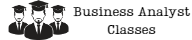# Exploratory Data Analysis: Finding Patterns and Anomalies in Large Datasets

Data is the new oil. We are living in a world where data is being generated at an unprecedented pace. Every day, we generate a huge amount of data, and this data is growing exponentially. This data is generated from various sources such as social media, IoT devices, sensors, and many other sources. The data generated is unstructured, and it’s difficult to extract meaningful insights from it. This is where exploratory data analysis (EDA) comes into play.Exploratory data analysis is a technique used by data scientists to analyze and summarize datasets. It helps in finding patterns, relationships, and anomalies in the data. In this article, we will discuss how exploratory data analysis can be used to find patterns and anomalies in large datasets.

## What is Exploratory Data Analysis?

Exploratory data analysis is a process of analyzing and summarizing datasets to find patterns, relationships, and anomalies. It is an iterative process that involves visualizing the data, cleaning the data, transforming the data, and modeling the data. The goal of EDA is to gain insights into the data and to formulate hypotheses that can be tested with statistical models.

EDA is a crucial step in the data analysis process as it helps in understanding the data and identifying potential problems. It provides a foundation for further analysis and modeling. EDA is not a substitute for formal statistical inference but rather a way to explore the data and formulate hypotheses.

## Why is Exploratory Data Analysis Important?

Exploratory data analysis is important because it helps in finding patterns, relationships, and anomalies in the data. It helps in understanding the data and identifying potential problems. EDA provides a foundation for further analysis and modeling. EDA is not a substitute for formal statistical inference but rather a way to explore the data and formulate hypotheses.

EDA is important because it helps in identifying potential problems with the data. For example, missing data, outliers, and data entry errors can be identified during the EDA process. Identifying these problems early can save time and resources later in the analysis.

## Steps in Exploratory Data Analysis

The steps in exploratory data analysis include:

### 1. Data Collection

Data collection is the first step in the EDA process. The data can be collected from various sources such as databases, files, and APIs.

### 2. Data Cleaning

Data cleaning involves identifying and correcting errors and inconsistencies in the data. This step is crucial as it ensures that the data is accurate and reliable.

### 3. Data Transformation

Data transformation involves converting the data into a format that can be easily analyzed. This step can involve aggregating data, scaling data, and normalizing data.

### 4. Data Visualization

Data visualization involves creating visual representations of the data. This step helps in identifying patterns and relationships in the data.

### 5. Data Modeling

Data modeling involves creating statistical models to test hypotheses about the data. This step helps in understanding the data and making predictions.

## Techniques Used in Exploratory Data Analysis

There are various techniques used in exploratory data analysis. Some of the techniques include:

### 1. Descriptive Statistics

Descriptive statistics involve summarizing and describing the data using measures such as mean, median, and mode.

### 2. Data Visualization

Data visualization involves creating visual representations of the data using charts, graphs, and plots.

### 3. Correlation Analysis

Correlation analysis involves examining the relationship between two variables in the data.

### 4. Clustering Analysis

Clustering analysis involves grouping data points into clusters based on their similarity.

### 5. Principal Component Analysis

Principal component analysis involves transforming the data into a new set of variables that are uncorrelated.

## Conclusion

Exploratory data analysis is a crucial step in the data analysis process. It helps in finding patterns, relationships, and anomalies in the data. EDA is not a substitute for formal statistical inference but rather a way to explore the data and formulate hypotheses. In this article, we discussed the steps in EDA and the techniques used in EDA. By performing EDA, data scientists can gain insights into the data and make better decisions based on the insights gained.

### Try some of our classes

Enter your email and we’ll send you some samples of our favorite classes.

#### Course Categories

Quisque velit nisi, pretium ut lacinia in, elementum id enim.